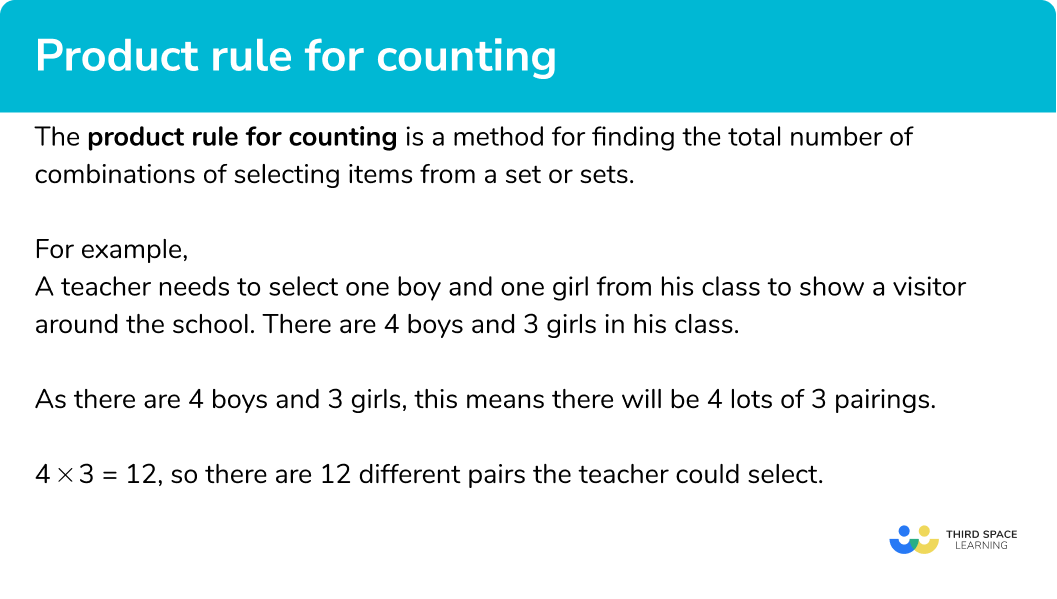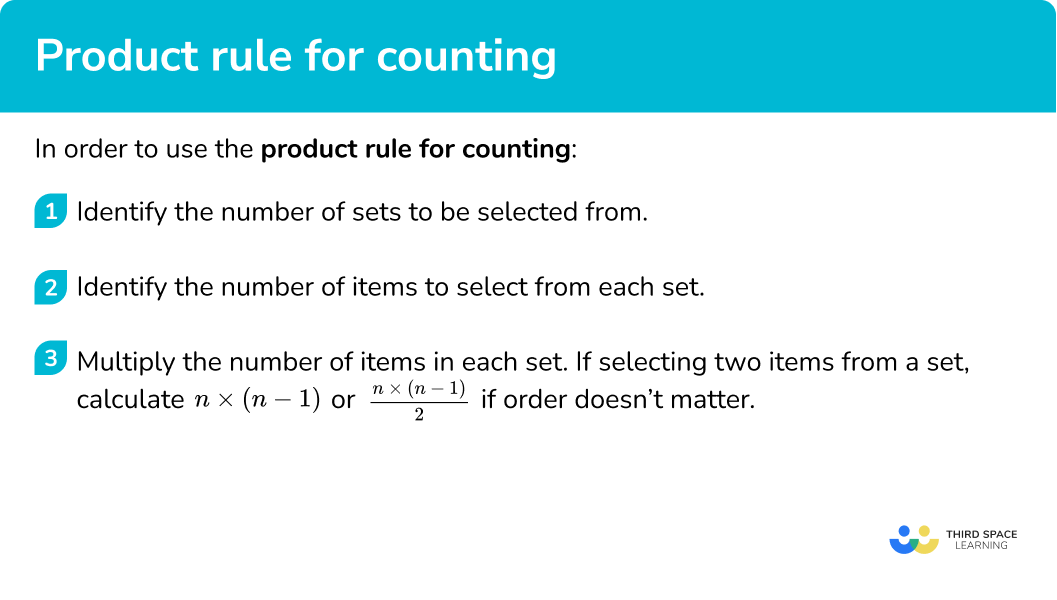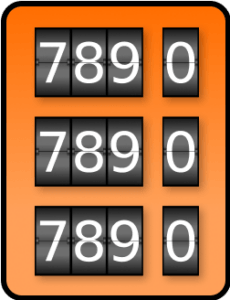GCSE Maths Probability Combined Events

Product Rule For Counting

# Product Rule For Counting

Here we will learn about the product rule for counting, including selecting from one set and selecting from multiple sets.

There are also counting strategies worksheets based on Edexcel, AQA and OCR exam questions, along with further guidance on where to go next if you’re still stuck.

## What is the product rule for counting?

In combinatorics the product rule for counting is a method for finding the total number of ways of selecting items from a set or sets. This is part of the new GCSE specifications. Finding or listing the total number of combinations is also known as enumeration.

For example,

A teacher needs to select one boy and one girl from his class to show a visitor around the school.

There are 4 boys and 3 girls in his class.

The number of different pairs of children can be calculated by thinking about how many different options there are for each boy.

Each boy could be paired with 3 girls.

As there are 4 boys, this means there will be 4 lots of 3 pairings.

4 \times 3 = 12, so there are 12 different pairs (permutations) the teacher could select.

If the boys are called A, B, C and D and the girls are called X, Y, Z the pairings would be:

AXAYAZ

BXBYBZ

CXCYCZ

DXDYDZ

If the teacher decided to just select two girls from his class we would need to think more carefully.

There would be 3 options for the first choice, and then 2 options for the second choice. However, if calculating 3 \times 2 would give double the amount of options as it would include each pair of girls twice and the order of selecting the girls doesn’t matter.

So there are \frac{3\times 2}{2}=3 pairs of girls to select.

The possible combinations would be, XY (same as YX), XZ (same as ZX) and YZ (same as ZY).

### What is the product rule for counting?## How to use the product rule for counting

In order to use the product rule for counting:

1. Identify the number of sets to be selected from.
2. Identify the number of items to select from each set.
3. Multiply the number of items in each set. If selecting two items from a set, calculate n\times \left( n-1 \right) or \frac{n\times \left( n-1 \right)}{2} if order doesn’t matter.

### Explain how to use the product rule for counting## Related lessons on combined events

Product rule for counting is part of our series of lessons to support revision on combined events. You may find it helpful to start with the main combined events lesson for a summary of what to expect, or use the step by step guides below for further detail on individual topics. Other lessons in this series include:

## Product rule for counting examples

### Example 1: selecting a pair from two different sets

Arthur has been told he can select a packet of crisps and a drink as part of a meal deal.

There are 7 different flavours of crisps and 11 different drinks.

How many possible outcomes could Arthur select?

1. Identify the number of sets to be selected from.

There are two sets to select from, crisps and drinks.

2Identify the number of items to select from each set.

There are 7 different crisps flavours and 11 different drinks.

3Multiply the number of items in each set. If selecting two items from a set, calculate n\times \left( n-1 \right) or \frac{n\times \left( n-1 \right)}{2} if order doesn’t matter.

7\times 11=77

There are 77 different pairs that Arthur could select.

### Example 2: selecting from multiple sets

Bella is clothes shopping and can select a t-shirt, a pair of jogging bottoms and hoodie as part of a mix and match offer.

There are 4 different colours of t-shirts, 5 colours for the jogging bottoms and 3 colours for the hoodie.

What is the number of outcomes Bella could select?

Identify the number of sets to be selected from.

Identify the number of items to select from each set.

Multiply the number of items in each set. If selecting two items from a set, calculate n\times \left( n-1 \right) or \frac{n\times \left( n-1 \right)}{2} if order doesn’t matter.

### Example 3: selecting a pair from one set when order matters

A teacher is trying to pick two students to participate in a quiz. They must also decide which student will go first and which will go second in a round of the quiz. If there are 24 students to choose from, how many different options does the teacher have?

Identify the number of sets to be selected from.

Identify the number of items to select from each set.

Multiply the number of items in each set. If selecting two items from a set, calculate n\times \left( n-1 \right) or \frac{n\times \left( n-1 \right)}{2} if order doesn’t matter.

### Example 4: selecting a pair from one set when order doesn’t matter

15 teams in a league must play each other once. How many games in total will be played?

Identify the number of sets to be selected from.

Identify the number of items to select from each set.

Multiply the number of items in each set. If selecting two items from a set, calculate n\times \left( n-1 \right) or \frac{n\times \left( n-1 \right)}{2} if order doesn’t matter.

### Example 5: selecting two pairs from two different sets

There are 8 boys and 10 girls to select from. How many different combinations are possible?

Identify the number of sets to be selected from.

Identify the number of items to select from each set.

Multiply the number of items in each set. If selecting two items from a set, calculate n\times \left( n-1 \right) or \frac{n\times \left( n-1 \right)}{2} if order doesn’t matter.

### Common misconceptions

• Forgetting to multiply by one less if selecting from a single set

A common error is to forget that when selecting two items from a set, the second choice is from a set reduced in size by one. We must assume that the first choice is not replaced unless the question specifically states that it is.

• Not knowing when order matters or not

Some students find it confusing to know when the order of a pair of items selected from a single set matters. If there are n items in the set and items are labelled A, B, C, …. calculating n\times \left( n-1 \right) will include the pairs AB and BA.

If the question was about teams playing each other only once, we would need divide this value by 2 to give the correct number of pairings, if, however, the teams needed to play each other twice the n\times \left( n-1 \right) would be correct.

### Practice product rule for counting questions

1. There are 7 starters and 12 main courses to choose from at a restaurant. How many different ways are there of choosing a starter and main course?

77847266For each 7 starters there are 12 possible main course choices, so calculate 7\times 12=84.

2. There are 6 starters, 8 main courses and 4 desserts to choose from at a restaurant. How many different ways are there of choosing a starter, main course and dessert?

1268018192For each 6 starters there are 8 possible main course choices and then 4 dessert choices, so calculate 6\times 8\times 4=192.

3. A teacher must choose two children from his class to show a visitor around the school. There are 22 children in his class. How many options does the teacher have to choose from?

462484231242Order doesn’t matter so calculate 22\times 21=462 and then half this answer 462\div 2=231.

4. A hockey league has 10 teams. Each team must play each other twice, home and away. How many games will be played in total?

901004550Order matters so calculate 10\times 9=90.

There are 6 boys and 7 girls to select from. How many different combinations are possible?

17641260882630There are 6 choices for head boy and 7 for head girl. Then there are 5 choices for deputy head boy and 6 for deputy head girl.

6\times 7\times 5\times 6=1260

6. A meal deal consists of one drink and one sandwich. There are 9 drinks and x sandwiches to choose from. Which of the following cannot not be the amount of ways to choose a meal deal.

728111699The amount of ways would be 9\times x, therefore it must be a multiple of 9.

### Product rule for counting GCSE questions

1. There are 14 boys and 16 girls in a class. The teacher must choose one boy and one girl to be class representatives.

How many different ways are there of choosing one boy and one girl?

(2 marks)

14\times 16

(1)

224

(1)

2. A combination lock has 3 dials, each with the digits 0 to 9.Sarah chooses her combination using a set of rules.

The first number will be square.

The second number will be prime.

The third number will be odd.

(a) How many different combinations could Sarah choose?

(b) Sarah decides to include 0 as an option to each of the three numbers and says this will double the number of combinations.

(3 marks)

(a)

Sight of a list of square or prime numbers 1, 4, 9 OR 2, 3, 5, 7.

(1)

3\times 4\times 5

(1)

60

(1)

(b)

Yes, answer is now 4\times 5\times 6=120 .

(1)

3. A group of 8 people arrive for a meeting and shake each other’s hands. How many handshakes are there in total?

(2 marks)

8\times 7\div 2

(1)

28

(1)

## Learning checklist

You have now learned how to:

• Apply use of the product rule for counting

## Still stuck?

Prepare your KS4 students for maths GCSEs success with Third Space Learning. Weekly online one to one GCSE maths revision lessons delivered by expert maths tutors.

Find out more about our GCSE maths tuition programme.